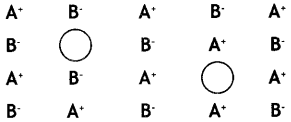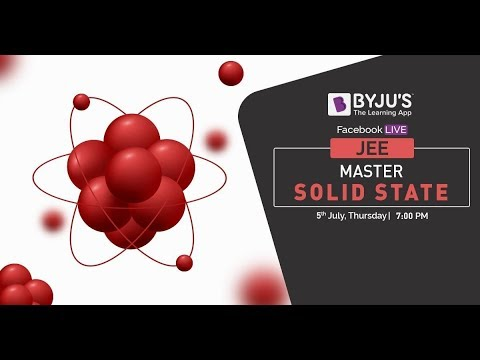# Solid State Questions

Solid is a state of matter in which the constituent particles are very closely packed together. Atoms, volume, ions, and other constituent particles can be found in a solid substance. Solid objects have a specific shape, mass, and volume due to strong and short interactions between their particles. Inside solids are classified into two types based on the order in which their particles are arranged. These are crystalline solids and amorphous solids.

 Definition: Solid-state chemistry, also known as materials chemistry, is the study of the synthesis, structure, and properties of solid phase materials, particularly but not exclusively non-molecular solids. As a result, it has a lot in common with solid-state physics, mineralogy, crystallography, ceramics, metallurgy, thermodynamics, materials science, and electronics. On the basis of the nature of order present in the arrangement of their constituent particles, solids can be classified as crystalline or amorphous.

## Solid State Chemistry Questions with Solutions

Q1. Schottky defect in crystals is observed when:

1. an ion leaves its normal site and occupies an interstitial site
2. an unequal number of cations and anions are missing from the lattice
3. density of the crystal is increased
4. an equal number of cations and anions are missing from the lattice

Correct Answer: (d) an equal number of cations and anions are missing from the lattice.

Solution: When an equal number of cations and anions are missing from the lattice, a Schottky defect is observed. As a result, the crystal remains neutral, for example, NaCl and the density of the crystal decreases.

Q2. Zn convert its melted state to its solid-state, it has hcp structure then find out a number of nearest atom:

1. 6
2. 8
3. 12
4. 4

Solution: hcp is a closely packed arrangement with hexagonal unit cells and a co-ordination number of 12.

Q3. With which one of the following elements silicon should be doped so as to give p-type of semiconductor?

1. Germanium
2. Arsenic
3. Selenium
4. Boron

Solution: When Si or Ge is doped with traces of elements from group 15, such as arsenic (As), n-type semiconductors are formed, whereas p-type semiconductors are formed when Si or Ge is doped with traces of elements from group 13, such as indium (In), boron.

Q4. Which point defect in crystals reduces crystal density?

Q5. Give reason:

(a) Why is Frenkel defect found in AgCl?

(b) What is the difference between phosphorus-doped and gallium doped silicon semiconductors?

(a) AgCl has a Frenkel defect because Ag+ is small enough to fit into interstitial vacant positions. As a result of the large size difference between the ions Ag+ and Cl, Ag+ occupies voids.

(b) Phosphorus-doped semiconductors produced n-type semiconductors, whereas gallium-doped silicon produced p-type semiconductors.

Q6. Tungsten crystallises in the body-centred cubic unit cell. If the edge of the unit cell is 316.5 pm, what is the radius of the tungsten atom?

If a is the edge length of the bcc unit cell, then the radius of an atom,

$$\begin{array}{l}r=\frac{\sqrt{3}}{4}a\end{array}$$

Here, a = 316.5 pm

$$\begin{array}{l}r=\frac{\sqrt{3}}{4}\times 316.5\end{array}$$

r = 137.04 pm

Q7. What are diamagnetic substances?

Answer. Diamagnetic substances are those that are only weakly repelled by an external magnetic field. For example, copper, bismuth, and so on.

Q8. The density of copper metal is 8.95 g cm–3. If the radius of copper atom is 127.8 pm, is the copper unit cell simple cubic, body centred cubic or face-centred cubic? (At mass of Cu=63.54g mol–1 and NA = 6.02×1023 mol–1)

Density, d = (Z×M)/(a3×NA)

Assuming fcc lattice for copper

$$\begin{array}{l}a=2\sqrt{2}r\end{array}$$

= 2 × 1.414 × 127.8 × 10–10 cm

∴a3 = (2 × 1.414 × 127.8 × 10–10 cm)3

= 4.721 × 10–23 cm3

$$\begin{array}{l}d=\frac{4\times 63.54}{4.721\times 10^{-23}\times 6.02\times 10^{23}}\end{array}$$

= 8.94 g cm–3

Since the density is same as observed, the lattice is fcc lattice.

Q9. Distinguish between crystalline solid and amorphous solid.

Answer. The difference between crystalline solid and amorphous solid are as follows-

CRYSTALLINE SOLIDS AMORPHOUS SOLIDS
Atoms are arranged in regular 3 dimension They do not have regular arrangement
Sharp melting point No particular melting point
Anisotropic Isotropic
True solid Pseudo solid
Symmetrical Unsymmetrical
More rigid Less rigid
Long range order Short range order
Example: Potassium nitrate, copper Example: Cellophane, polyvinyl chloride

Q10. Find the number of atoms in fcc unit cell.

Answer. There are 8 atoms at each of the 8 corners and 6 atoms at each of the 6 face centres in this unit cell. Since each corner contributes 1/8th of an atom to the unit cell, the number of atoms = 1/8 × 8 = 1.

Since each face centre contributes half an atom to the unit cell, the number of atoms = 1/2 × 6 = 3.

∴ The total number of atoms in a fee unit cell is 1 + 3 = 4.

As a result, the volume of the unit cell equals the volume of four atoms.

Q11. Calculate the packing efficiency for bcc lattice.

$$\begin{array}{l}a=\frac{4r}{\sqrt{3}}\end{array}$$

The volume of the unit cell is a3

$$\begin{array}{l}a^{3}=\left ( \frac{4r}{\sqrt{3}} \right )^{3}=\frac{64r^{3}}{3\sqrt{3}}\end{array}$$

Volume occupied by 1 atom is

$$\begin{array}{l}\frac{4}{3}\Pi r^{3}\end{array}$$

A bcc unit cell has 2 atoms per unit cell.

Therefore, the volume occupied by 2 atoms is

$$\begin{array}{l}2\times \frac{4}{3}\Pi r^{3}=\frac{8}{3}\Pi r^{3}\end{array}$$

Packing efficiency = Volume occupied by atoms in a unit cell

Total volume of unit cell ✕ 100

Packing efficiency =

$$\begin{array}{l}\frac{\frac{8}{3}\Pi r^{3}}{\frac{64r^{3}}{3\sqrt{3}}}\times 100\end{array}$$

Packing efficiency = 68.04%

1. What type of semiconductor is obtained when silicon is doped with boron?
2. What type of magnetism is shown in the following alignment of magnetic moments?
↑ ↑ ↑ ↑ ↑ ↑ ↑
3. What type of point defect is produced when AgCI is doped with CdCl2?

1. When silicon is doped with boron, which has three valence electrons, the bonds formed produce electron deficient sites known as holes. Under the influence of the applied electric field, one electron from a neighbouring atom moves to fill the hole but creates a new hole at the same time. As a result, the electrical conductance is caused by the movement of positive holes. As a result, this type of semi-conductor is known as a p-type semi-conductor.
2. The magnetism depicted in the magnetic moment alignment is Ferromagnetic.
3. When CdCl2 is added to AgCl, it introduces an impurity defect. To maintain electrical neutrality, one Cd2+ ion will replace two Ag+ ions. Cd2+ ion will occupy one of the Ag+ positions, leaving the other unoccupied. As a result, a hole similar to the Schottky defect is formed.

Q13. What is point defects. Describe the two types of point defects?

Answer. Point defects are defects in crystals that occur around an atom or particle. These flaws can only be found at or near a single lattice point. They do not extend in any dimension of space. These are the tiniest defects that can exist in any crystalline solid material. Point flaws appear when:

• One or more crystal atoms are missing from their corresponding lattice site.
• The atom(s) is shifted from its corresponding lattice site to the crystal’s interstitial position.
• The interstitial position in the crystal lattice is occupied by a foreign atom or atoms.
• A foreign atom replaces the crystal’s original atom.

The two types of defects are-

1. Stoichiometric Defects: These are point defects in a crystal that do not affect the solid’s stoichiometry. These compounds follow the laws of definite proportions, constant composition, and mass conservation. They’re also known as intrinsic or thermodynamic flaws.
2. Non-Stoichiometric Defect: These defects are caused by changes in the cation and anion ratios caused by the addition or removal of ions from the crystal.

Q14. Examine the given defective crystal1. What type of stoichiometric defect is shown by the crystal?
2. How is the density of the crystal affected by this defect?
3. What type of ionic substances show such defects?

1. Schottky defect
2. Density of the crystal decreases.
3. Crystals having

(i) high coordination number and

(ii) ions (cations and anions) of almost similar sizes. For example KCl, KBr.

1. Differentiate between Schottky defect and Frenkel defect?
2. Explain:

(i) Why is Frenkel defect not found in pure alkali metal halides?

(ii) Zinc oxide is white but it turns yellow on heating.

(iii) CaCl2 will introduce Schottky defect when added to AgCl crystal.

Schottky Defect Frenkel Defect
In Schottky defect the difference in size between cation and anion is small. Frenkel defect contains ionic crystals where the anion is larger than the cation.
Both anion and cation leave the solid crystal. Usually the smaller ion cation leaves its original lattice structure.
Atoms permanently leave the crystal. Atoms remain within the solid crystal.
There is formation of two vacancies. Only one vacancy is created.
The density of the solid decreases. Density remains the same.

(i)The Frenkel defect does not exist in pure alkali metal halides because the ions are too large to enter the interstitial sites.

(ii) When ZnO is heated, it loses oxygen in the following ways:

ZnO → Zn2+ + ½ O2 +2e

To maintain electrical neutrality, Zn2+ ions are entrapped in the interstitial sites, while electrons are entrapped in neighbouring interstitial sites. As a result, there is an excess of metal defect. The colour is yellow due to the presence of free electrons in the interstitial sites.

(iii) When CaCl2 is added to AgCl, it introduces an impurity defect. To maintain electrical conductivity, one Ca2+ ion will replace two Ag+ ions. Ca2+ ion will occupy one of the Ag+ positions, leaving the other as a hole. As a result, a hole similar to the Schottky defect is formed.

## Practise Questions on Solid State

Q1. Which of the following is an amorphous solid?

1. Copper sulphate
2. Magnesium
3. Tar
4. Diamond

Q2. Sodium crystallizes in bcc structure. If the edge length of unit cell is 4.3 ✕ 10-8 cm, the radius of Na atom is ____.

1. 1.86 ✕ 10-8
2. 1.52 ✕ 10-8
3. 2.15 ✕ 10-8
4. 4.3 ✕ 10-8

Q3. Aluminium crystallises in a fcc structure. The atomic radius of the metal is 125 pm. What is the length of the side of unit cell of the metal?

Q4. Explain with a diagram, the vacancy defect.

Q5. Calculate the packing efficiency of a metal crystal for a simple cubic lattice.

Click the PDF to check the answers for Practice Questions.

## Recommended Videos

#### Solid State#### Types of Solids